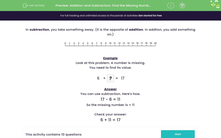# Find the Missing Number Using Addition or Subtraction

In this worksheet, students will solve missing number problems using subtraction (0-20).Key stage:  KS 1

Curriculum topic:   Number: Addition and Subtraction

Curriculum subtopic:   Solve Add/Subtract Problems to 20

Popular topics:   Subtraction worksheets, Addition worksheets

Difficulty level:#### Worksheet Overview

In this activity, we are going to look at how we can use subtraction to work out the missing number in an addition sentence.

Subtraction is the inverse of addition. Let's look at what this means.

6 + 7 = 13

We know we can switch the numbers over in an addition sentence and it gives the same answer:

7 + 6 = 13

With the inverse, it means we can use the same numbers and make subtraction sentences.

There is one rule to remember: when we make a subtraction sentence, the biggest number always goes first.

Here are the subtraction sentences for the above example:

13 - 7 = 6

13 - 6 = 7

Example 1

Look at this problem. A number is missing.

You need to find its value by using the inverse.

 6 + ? = 17

So we need to use subtraction to find the missing number.

All we need to do is make sure we put the biggest number first and make a subtraction sentence using the numbers we have:

17 - 6 = ?

Now we just need to work out 17 - 6 =We need to start on 17 and count backwards 6.

This gives us 11.

17 - 6 = 11

Now we have found out the missing number we can put it back into the missing spot!

 6 + 11 = 17

Example 2

Look at this problem. A number is missing.

You need to find its value by using the inverse.

 ? - 5 = 17

This number sentence has used subtraction, so we need to use the inverse (which is addition) to find the missing number.

We use the numbers we already have and make an addition sentence. Remember, in addition the numbers can be in any order!

It is a good idea to start with the biggest number so that you have fewer numbers to add on:

17 + 5 = ?

Now we just need to work this out. Start on 17 and add on 5.

This gives us 22.

Now we have worked out the missing number, we test it in the original number sentence to check it works!

 22 - 5 = 17

It's simple, isn't it?### What is EdPlace?

We're your National Curriculum aligned online education content provider helping each child succeed in English, maths and science from year 1 to GCSE. With an EdPlace account you’ll be able to track and measure progress, helping each child achieve their best. We build confidence and attainment by personalising each child’s learning at a level that suits them.

Get started#### Popular Maths topics

••••